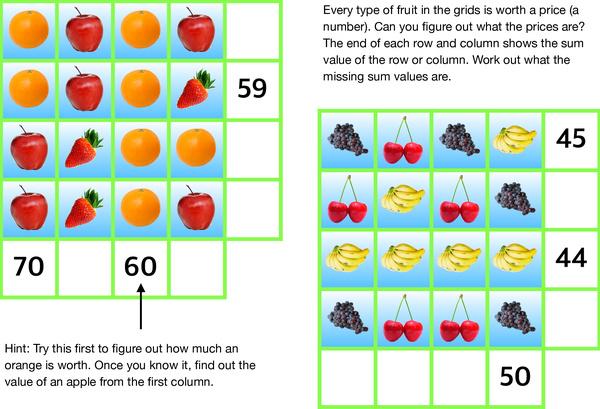# A fruity challenge using simple algebraAlgebra is an important part of mathematics, with symbols and rules for manipulating those symbols to solve a problem. Elementary algebra is essential for studies of many fields such as mathematics, science, engineering, economics, and it is also necessary for daily life. Secondary school students start learning elementary algebra, and also apply in understanding arithmetics. This worksheet presents two challenges with grids of various fruits, which can be solved by applying simple algebra. Each type of fruit in the grids is worth some price (a number). As the hint indicated, the value of each type of fruit can be figured out one by one. With this information, the missing sum values at the end of each row and column could also be worked out. This is a game for kids to practice operations of addition and subtraction, and even of multiplication and dividend.